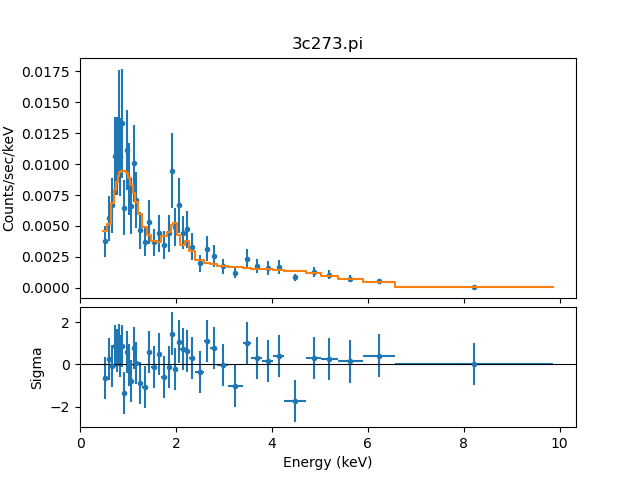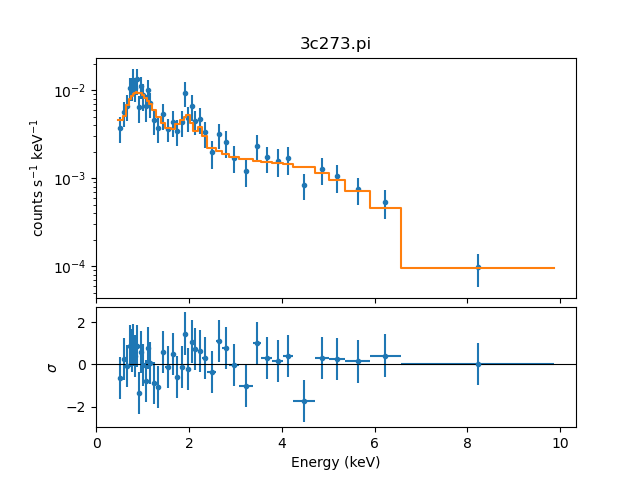# How can I modify a single plot in a display containing multiple plots?

Plotting in Sherpa is done with a combination of Sherpa functions to create the plot (such as plot_data, plot_fit_delchi, reg_proj, and contour_model) and configure the plot (for example set_ylog and get_data_plot_prefs), and matplotlib commands to augment or change the resulting visualizations.

plot_fit_resid and plot_fit_delchi are examples of Sherpa commands which create multiple "plot areas".

sherpa> plot_fit_delchi(xlog=True)


### The plot_fit_delchi output from Sherpa### The plot_fit_delchi output from Sherpa

The xlog preference was over-ridden (set to True) in the plot_fit_delchi call, so the X axis is drawn with a logarithmic scale for both plots. This capability is new to Sherpa in CIAO 4.12.

The two plot areas can be accessed using the axes field of the object returned by the matplotlib gcf routine:

sherpa> fig = plt.gcf()
sherpa> len(fig.axes)
2


The top plot is the first element and the bottom plot the second element, so to change the label of the Y axis of the top and bottom plots, and the scaling of the top plot, we can say:

sherpa> ax1, ax2 = fig.axes
sherpa> ax1.set_ylabel(r'counts s$^{-1}$ keV$^{-1}$')
sherpa> ax1.set_yscale('log')
sherpa> ax2.set_ylabel(r'$\sigma$')


and then, to add more space to the Y axis

sherpa> plt.subplots_adjust(left=0.15)


### Changing the plot